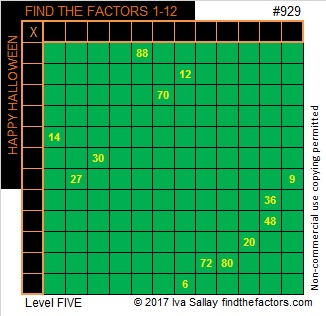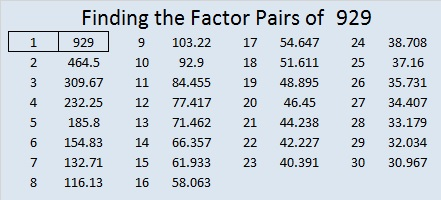# 929 Little Green Monster

Here’s a little green monster just in time for Halloween. It’s a level 5 so it might be a little scary. Just don’t write any of the factors in the first column or top row unless you know for sure that factor belongs where you are putting it. Use logic and not guessing, and you’ll handle this little green monster just fine.Print the puzzles or type the solution on this excel file: 12 factors 923-931

929 is the sum of nine consecutive prime numbers:
83  + 89 + 97 + 101 + 103 + 107 + 109 + 113 + 127 = 929

23² + 20² = 929, so 929 is the hypotenuse of a Pythagorean triple:
129-920-929 which is 23² – 20², 2(23)(20), 23² + 20²

Obviously 929 is a palindrome in base 10.

It is also palindrome 131 in BASE 29 because 1(29²) + 3(29) + 1(1) = 929.

• 929 is a prime number.
• Prime factorization: 929 is prime.
• The exponent of prime number 929 is 1. Adding 1 to that exponent we get (1 + 1) = 2. Therefore 929 has exactly 2 factors.
• Factors of 929: 1, 929
• Factor pairs: 929 = 1 × 929
• 929 has no square factors that allow its square root to be simplified. √929 ≈ 30.4795013

How do we know that 929 is a prime number? If 929 were not a prime number, then it would be divisible by at least one prime number less than or equal to √929 ≈ 30.4. Since 929 cannot be divided evenly by 2, 3, 5, 7, 11, 13, 17, 19, 23, or 29, we know that 929 is a prime number.